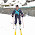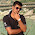Drilling Hole Volume Calculation

In Drilling,Hole volume calculations include :-
1. Drill string Capacity or Casing capacity calculation or pipe capacity
2. Pipe displacement calculation
3. Annular volume calculation
These calculation are important as they gives us:-
• Volume of drilling mud in hole
• Volume of drilling mud required to displace the cement into the annulus
• To calculate gain or loss
• To calculate the height of influx if its volume is know
• To calculate Lag time(it is the time required by the cuttings after they are drilled to reach the surface)
Drill string Capacity or Casing capacity calculation- includes calculating the inside volume of drill string or Casing
Pipe Volume(bbl) = ID2*L/1029.4
where ID = internal diameter in inches, L= length in feet
Note:- Pipes with different internal diameter will have different pipe capacity and should be calculated separately.

Pipe displacement:- It is the metal volume of pipe.
Pipe displacement(bbl) = (OD2-ID2)*L/1029.4
where OD = outter diameter in inches

Example:-
Pipe Capacity:-
Drill pipe Volume = ID2*L/1029.4
=2.672*1000/1029.4
=7 bbl

Drill Collar Volume=  2.252*200/1029.4
= 1 bbl

Total pipe volume =Drill pipe +Drill collar volume
= 7+1
=8 bbl

Pipe Displacement:-
Drill pipe Displacement  =  (OD2-ID2)*L/1029.4
= (3.52-2.672)*1000/1029.4
= 5 bbl

Drill Collar Displacement=  (52-2.252)*200/1029.4
= 4 bbl

Total pipe Displacement =Drill pipe displacement+Drill collar displacement
=5+4
=9 bbl

1.Sir, say something about mud losses calculation........

1.refer to recent post
http://petroleumpro.blogspot.com/2017/06/Lost-circulation.html

2.3.Profundity Stops are an unquestionable requirement for ventures with tedious exhausting so as to keep consistency. Search for a drill press with the "Simple to-Stop" drill include. https://my-drill-press.jimdofree.com/